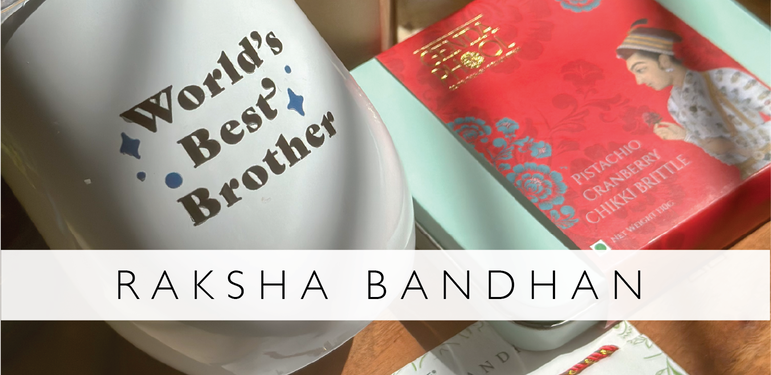# RakhiRs. 2,625.00

Rs. 2,100.00

Rs. 1,225.00

Rs. 700.00

Rs. 350.00

Rs. 350.00

Rs. 350.00

Rs. 350.00

Rs. 350.00

Rs. 350.00

Rs. 531.00

Rs. 590.00

Rs. 590.00

Rs. 472.00

Rs. 350.00

Rs. 350.00

Rs. 350.00

Rs. 350.00

Rs. 350.00

Rs. 350.00

Rs. 350.00

Rs. 200.00

Rs. 175.00

Rs. 175.00

Rs. 175.00

Rs. 175.00

Rs. 599.00

Rs. 599.00